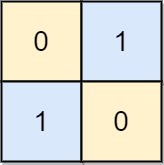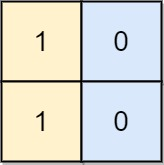## Algorithm

Problem Name: 782. Transform to Chessboard

You are given an `n x n` binary grid `board`. In each move, you can swap any two rows with each other, or any two columns with each other.

Return the minimum number of moves to transform the board into a chessboard board. If the task is impossible, return `-1`.

A chessboard board is a board where no `0`'s and no `1`'s are 4-directionally adjacent.

Example 1:```Input: board = [[0,1,1,0],[0,1,1,0],[1,0,0,1],[1,0,0,1]]
Output: 2
Explanation: One potential sequence of moves is shown.
The first move swaps the first and second column.
The second move swaps the second and third row.
```

Example 2:```Input: board = [[0,1],[1,0]]
Output: 0
Explanation: Also note that the board with 0 in the top left corner, is also a valid chessboard.
```

Example 3:```Input: board = [[1,0],[1,0]]
Output: -1
Explanation: No matter what sequence of moves you make, you cannot end with a valid chessboard.
```

Constraints:

• `n == board.length`
• `n == board[i].length`
• `2 <= n <= 30`
• `board[i][j]` is either `0` or `1`.

## Code Examples

### #1 Code Example with Javascript Programming

```Code - Javascript Programming```

``````
const movesToChessboard = function (b) {
let N = b.length,
rowSum = 0,
colSum = 0,
rowSwap = 0,
colSwap = 0;
for (let i = 0; i < N; ++i)
for (let j = 0; j < N; ++j)
if ((b ^ b[i] ^ b[j] ^ b[i][j]) === 1) return -1;
for (let i = 0; i < N; ++i) {
rowSum += b[i];
colSum += b[i];
if (b[i] === i % 2) rowSwap++;
if (b[i] === i % 2) colSwap++;
}
if (rowSum !== ((N / 2) >> 0) && rowSum !== ((N + 1) / 2)>>0 ) return -1;
if (colSum !== ((N / 2) >> 0) && colSum !== ((N + 1) / 2)>>0 ) return -1;
if (N % 2 === 1) {
if (colSwap % 2 === 1) colSwap = N - colSwap;
if (rowSwap % 2 === 1) rowSwap = N - rowSwap;
} else {
colSwap = Math.min(N - colSwap, colSwap);
rowSwap = Math.min(N - rowSwap, rowSwap);
}
return (colSwap + rowSwap) / 2;
};
``````
Copy The Code &

Input

cmd
board = [[0,1,1,0],[0,1,1,0],[1,0,0,1],[1,0,0,1]]

Output

cmd
2

### #2 Code Example with Python Programming

```Code - Python Programming```

``````
class Solution:
def movesToChessboard(self, b):
N = len(b)
if any(b ^ b[i] ^ b[j] ^ b[i][j] for i in range(N) for j in range(N)): return -1
if not N // 2 <= sum(b) <= (N + 1) // 2: return -1
if not N // 2 <= sum(b[i] for i in range(N)) <= (N + 1) // 2: return -1
col = sum(b[i] == i % 2 for i in range(N))
row = sum(b[i] == i % 2 for i in range(N))
if N % 2:
if col % 2: col = [col, N - col][col % 2]
if row % 2: row = N - row
else:
col = min(N - col, col)
row = min(N - row, row)
return (col + row) // 2
``````
Copy The Code &

Input

cmd
board = [[0,1,1,0],[0,1,1,0],[1,0,0,1],[1,0,0,1]]

Output

cmd
2